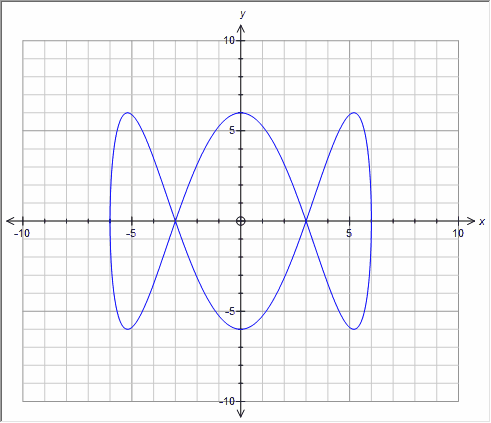﻿ Parametric

# Parametric

Top  Previous  Next

Parametric curves are entered as two equations in terms of t - separated by a semicolon (see regional issues).

x = 6cos t ;  y = 6sin 3tDefault Domains

Cartesian and Inverse Cartesian functions have an "implied domain" - the bounds of the axes you have drawn the function on.  If you have not explicitly set a domain, the graph tool can automatically determine a suitable domain from the axes it is using.  For parametric graphs, there is not a natural domain that can be easily determined.  To counter this problem, the graph tool uses a default domain for parametric graphs.

## Parametric graphs                0 ≤ t ≤ 20

In most cases the default domains produce acceptable results.  Sometimes you may need to override the default domains in order to create the result you are after.

Regional Issues

If you use a comma as a decimal point (eg 3,2) you must use a colon to separate the two parts of a parametric equation.

x = 6cos t :  y = 6sin 3t